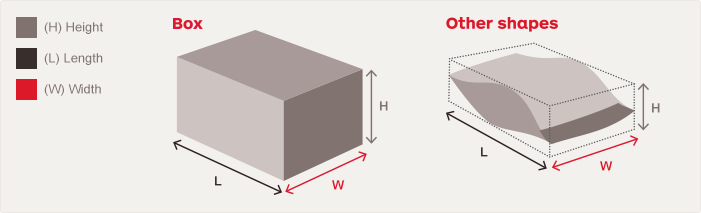Calculating parcel weight
Calculating the cubic weight of your parcel
The images below show you how to measure regular and irregular shaped parcels, and satchels.Handy tip: When measuring the length and width of your parcel, always use the maximum points.
The cubic weight is the parcel's volume in cubic centimetres, divided by 6,000. To work this out, use the formula below:
Height x length x width ÷ 6,000 = cubic weight

For example: 50cm X 30cm X 40cm
= 60,000cm3 ÷ 6,000
= 10kg cubic weight
If the actual weight of your parcel is 9kg but the cubic weight is 10kg, we'll charge you the cubic weight because it is greater. But if the parcel's actual weight is 16kg, we'll charge you the actual weight because it is greater than the cubic weight of 10kg.
Maximum weight and dimensions
The maximum weight of a single parcel that can be sent through ShopMate is 22kg. Individual parcels must not exceed the following dimensions:
• a maximum girth of 200cm
• no one side greater than 1.05m in length
• a dimension greater than 0.25 cubic metres
The formula for working out the girth of your overseas parcel is:
Width + height x 2 = girth

For example: 30cm + 20cm
= 50cm x 2
= 100cm girth

In the event that a parcel exceeds our weight or dimension restrictions, you will be notified and given the option to either pay for a return to the US merchant, or alternatively, pay \$5.00 AUD to have the item destroyed in the US.